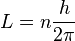# Bohr model facts for kids

Kids Encyclopedia Facts

In quantum mechanics, a branch of physics, the Bohr model is the model of the atom proposed by Niels Bohr. Bohr's model is useful only when discussing the behavior of a hydrogen atom. Bohr's model is extremely useful in describing the hydrogen emission spectrum.

## Electrons

### Energy Levels

The Bohr model says that the angular momentum of an electron within a hydrogen atom can only be integer multiples of a certain number.$L=n\frac{h}{2\pi}$

Where h is Planck's constant, π is pi, and n is the multiples (1,2,3,...). This formula says that an electron will only be found on the energy levels predicted by the formula, and not anywhere in between. This means that if an electron jumps from one energy level to the next, it will never be in between energy levels, but will instantaneously be transported from one level to the other.

### Light

Bohr's model is also useful because it explains the cause of light. Bohr agreed with classical theory that light has a wave-particle duality (meaning that it is made of both electromagnetic waves and particles called photons), but he said that light was given off when an electron in a hydrogen atom jumps from a higher energy level to a lower one. Basically, if an electron with x amount of energy goes to a lower state of energy and loses y energy, then a photon with energy y is emitted by the atom and either becomes light or some other form of radiation.

## Related pagesBohr model Facts for Kids. Kiddle Encyclopedia.